### Odds Ratio Estimation

Consider a dichotomous response variable with outcomes event and nonevent. Consider a dichotomous risk factor variable X that takes the value 1 if the risk factor is present and 0 if the risk factor is absent. According to the logistic model, the log odds function,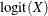, is given by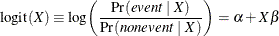The odds ratio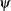is defined as the ratio of the odds for those with the risk factor (X = 1) to the odds for those without the risk factor (X = 0). The log of the odds ratio is given by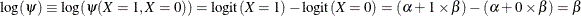In general, the odds ratio can be computed by exponentiating the difference of the logits between any two population profiles. This is the approach taken by the ODDSRATIO statement, so the computations are available regardless of parameterization, interactions, and nestings. However, as shown in the preceding equation for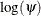, odds ratios of main effects can be computed as functions of the parameter estimates, and the remainder of this section is concerned with this methodology.

The parameter,, associated with X represents the change in the log odds from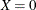to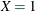. So the odds ratio is obtained by simply exponentiating the value of the parameter associated with the risk factor. The odds ratio indicates how the odds of the event change as you change X from 0 to 1. For instance,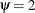means that the odds of an event when X = 1 are twice the odds of an event when X = 0. You can also express this as follows: the percent change in the odds of an event from X = 0 to X = 1 is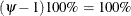.

Suppose the values of the dichotomous risk factor are coded as constants a and b instead of 0 and 1. The odds when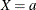become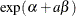, and the odds when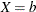become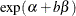. The odds ratio corresponding to an increase in X from a to b is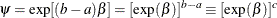Note that for any a and b such that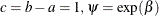. So the odds ratio can be interpreted as the change in the odds for any increase of one unit in the corresponding risk factor. However, the change in odds for some amount other than one unit is often of greater interest. For example, a change of one pound in body weight might be too small to be considered important, while a change of 10 pounds might be more meaningful. The odds ratio for a change in X from a to b is estimated by raising the odds ratio estimate for a unit change in X to the power of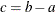as shown previously.

For a polytomous risk factor, the computation of odds ratios depends on how the risk factor is parameterized. For illustration, suppose that Race is a risk factor with four categories: White, Black, Hispanic, and Other.

For the effect parameterization scheme (PARAM=EFFECT) with White as the reference group (REF=’White’), the design variables for Race are as follows:

Design Variables

Race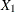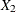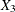Black

1

0

0

Hispanic

0

1

0

Other

0

0

1

White

–1

–1

–1

The log odds for Black is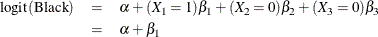The log odds for White is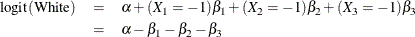Therefore, the log odds ratio of Black versus White becomes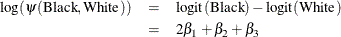For the reference cell parameterization scheme (PARAM=REF) with White as the reference cell, the design variables for race are as follows:

Design Variables

RaceBlack

1

0

0

Hispanic

0

1

0

Other

0

0

1

White

0

0

0

The log odds ratio of Black versus White is given by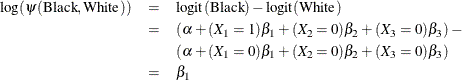For the GLM parameterization scheme (PARAM=GLM), the design variables are as follows:

Design Variables

Race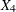Black

1

0

0

0

Hispanic

0

1

0

0

Other

0

0

1

0

White

0

0

0

1

The log odds ratio of Black versus White is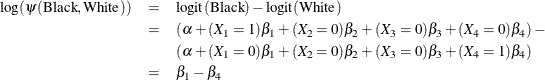Consider the hypothetical example of heart disease among race in Hosmer and Lemeshow (2000, p. 56). The entries in the following contingency table represent counts:

Race

Disease Status

White

Black

Hispanic

Other

Present

5

20

15

10

Absent

20

10

10

10

The computation of odds ratio of Black versus White for various parameterization schemes is tabulated in Table 58.11.

Table 58.11: Odds Ratio of Heart Disease Comparing Black to White

Parameter Estimates

PARAM=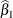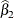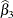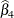Odds Ratio Estimates

EFFECT

0.7651

0.4774

0.0719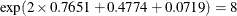REF

2.0794

1.7917

1.3863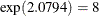GLM

2.0794

1.7917

1.3863

0.0000Since the log odds ratio () is a linear function of the parameters, the Wald confidence interval forcan be derived from the parameter estimates and the estimated covariance matrix. Confidence intervals for the odds ratios are obtained by exponentiating the corresponding confidence limits for the log odd ratios. In the displayed output of PROC LOGISTIC, the Odds Ratio Estimates table contains the odds ratio estimates and the corresponding 95% Wald confidence intervals. For continuous explanatory variables, these odds ratios correspond to a unit increase in the risk factors.

To customize odds ratios for specific units of change for a continuous risk factor, you can use the UNITS statement to specify a list of relevant units for each explanatory variable in the model. Estimates of these customized odds ratios are given in a separate table. Let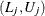be a confidence interval for. The corresponding lower and upper confidence limits for the customized odds ratio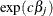are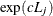and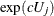, respectively (for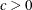), orand, respectively (for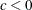). You use the CLODDS= option or ODDSRATIO statement to request the confidence intervals for the odds ratios.

For a generalized logit model, odds ratios are computed similarly, except k odds ratios are computed for each effect, corresponding to the k logits in the model.